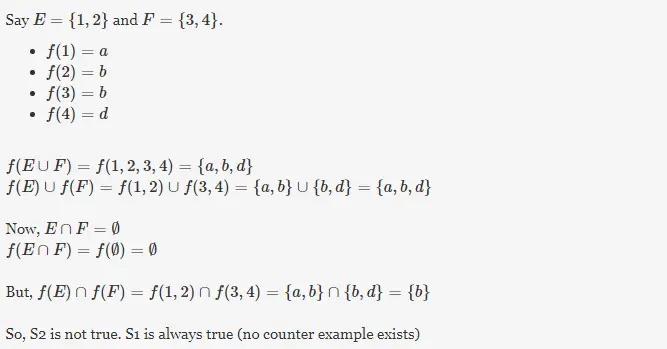# Gate CS-2001 Question Paper With Solutions

Q. 28 Let f: A→B be a function, and let E and F be subsets of A. Consider the following statements about images.

```S1: f (E ∪ F) = f (E) ∪ f (F)
S1: f (E ∩ F) = f (E) ∩ f (F)```

Which of the following is true about S1 and S2?

(A) Only S1 is correct

(B) Only S1 is correct

(C) Both S1 and S2 are correct

(D) None of S1 and S2 is correct